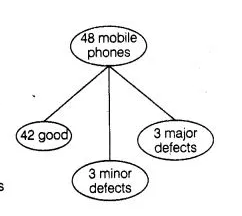# A lot consists of 48 mobile phones of which 42 are good,

Question:

A lot consists of 48 mobile phones of which 42 are good, 3 have only minor defects and 3 have major defects. Varnika will buy a phone, if it is good

but

the trader will only buy a mobile, if it has no major defect. One phone is selected at random from the lot. What is the probability that it is

(i)  acceptable to Varnika?

Solution:

Given, total number of mobile phones

$n(S)=48$(I) Let $E_{1}=$ Event that Varnika will buy a mobile phone

$=$ Varnika buy only, if it is good mobile

$\therefore \cap\left(E_{1}\right)=42$

$\therefore P\left(E_{1}\right)=\frac{n\left(E_{1}\right)}{n(S)}=\frac{42}{48}=\frac{7}{8}$

(ii) Let $E_{2}=$ Event that trader will buy only when it has no major defects

$=$ Trader will buy only 45 modiles

$\therefore \quad n\left(E_{2}\right)=45$

$\therefore \quad P\left(E_{2}\right)=\frac{n\left(E_{2}\right)}{n(S)}=\frac{45}{48}=\frac{15}{16}$